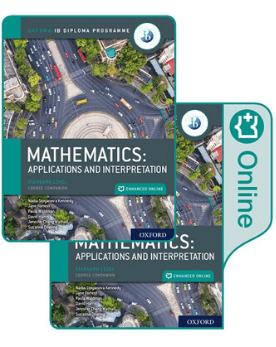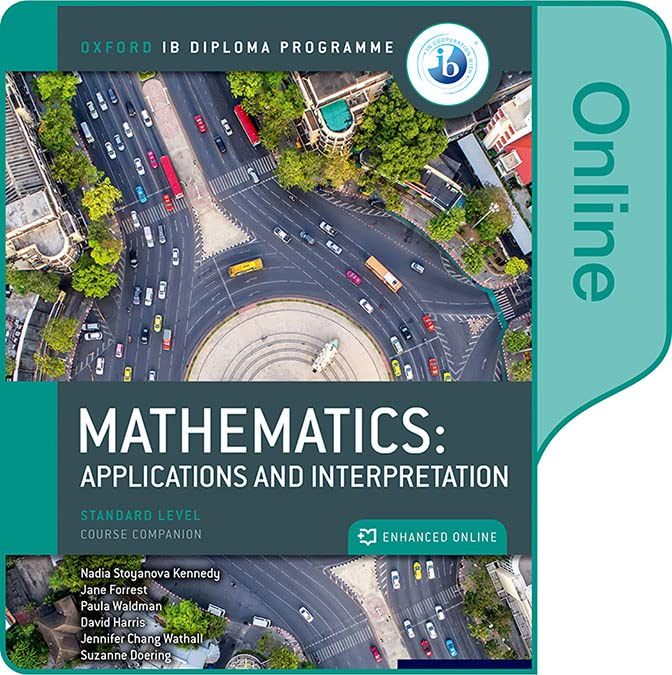# IB Mathematics: Applications and Interpretation, SL Course Companion

Regular price \$115.00
Sale price \$115.00 Regular price

Featuring a wealth of digital content, this concept-based Print and Enhanced Online Course Book Pack has been developed in cooperation with the IB to provide the most comprehensive support for the new DP Mathematics: applications and interpretation SL syllabus, for first teaching in September 2019.

### Features

• Address all aspects of the new DP Mathematics: applications and interpretation SL syllabus via an Enhanced Online Course Book Pack - made up of one full-colour, print textbook and one online textbook, including extensive teacher notes
• Ensure learners are ready to tackle each topic with targeted 'Prior Knowledge' worksheets, linked to 'Before You Start' summaries and exercises at the start of every chapter
• Deliver in-depth coverage of all topics through clear explanations and worked solutions, animated worked examples, differentiated exercises and worksheets, with answers provided
• Adopt a concept-based approach with conceptual lenses and microconcepts woven into every chapter, plus rich investigations that integrate factual and conceptual questions - leading to meaningful, content-specific conceptual understanding
• Deepen mathematical understanding via inquiry-based tasks that relate to the content of each chapter, 'international mindedness' features, regular links to Theory of Knowledge, and activities that target ATL skills
• Support students' development of a mathematical toolkit, as required by the new syllabus, with modelling and investigation activities presented in each chapter, including prompts for reflection, and suggestions for further study
• Thoroughly prepare students for IB assessment via in-depth coverage of course content, overviews of all requirements, exam-style practice questions and papers, and a full chapter supporting the new mathematical exploration (IA)
• Includes support for the most popular Graphic Display Calculator models
• This Online Course Book will be available on Oxford Education Bookshelf until 2029. Access is facilitated via a unique code, which is sent in the mail. The code must be linked to an email address, creating a user account.
• Access may be transferred once to an additional user.

Measuring space: accuracy and 2D geometry
1.1: Measurements and estimates
1.2: Recording measurements, significant digits and rounding
1.3: Measurements: exact or approximate?
1.4: Speaking scientifically
1.5: Trigonometry of right-angled triangles and indirect measurements
1.6: Angles of elevation and depression
Representing space: non-right angled trigonometry and volumes
2.1: Trigonometry of non-right triangles
2.2: Area of triangle formula. Applications of right and non-right angled trigonometry
2.3: Geometry: solids, surface area and volume
Representing and describing data: descriptive statistics
3.1: Collecting and organising univariate data
3.2: Sampling techniques
3.3: Presentation of data
3.4: Bivariate data
Dividing up space: coordinate geometry, lines, Voronoi diagrams
4.1: Coordinates, distance and midpoint formula in 2D and 3D
4.2: Gradient of lines and its applications
4.3: Equations of straight lines; different forms of equations
4.4: Parallel and perpendicular lines
4.5: Voronoi diagrams and toxic waste problem
Modelling constant rates of change: linear functions
5.1: Functions
5.2: Linear Models
5.3: Arithmetic Sequences
5.4: Modelling
Modelling relationships: linear correlation of bivariate data
6.1: Measuring correlation
6.2: The line of best fit
6.3: Interpreting the regression line
Quantifying uncertainty: probability, binomial and normal distributions
7.1: Theoretical and experimental probability

7.2: Representing combined probabilities with diagrams
7.3: Representing combined probabilities with diagrams and formulae
7.4: Complete, concise and consistent representations
7.5: Modelling random behaviour: random variables and probability distributions
7.6: Modelling the number of successes in a fixed number of trials
7.7: Modelling measurements that are distributed randomly
Testing for validity: Spearman's, hypothesis testing and x2 test for independence
8.1: Spearman's rank correlation coefficient
8.2: chi2 test for independence
8.3: chi2 goodness of fit test
8.4: The t-test
Modelling relationships with functions: power functions
9.3: Cubic models, power functions and direct and inverse variation
9.4: Optimisation
Modelling rates of change: exponential and logarithmic functions
10.1: Geometric sequences and series
10.2: Compound interest, annuities, amortization
10.3: Exponential models
10.4: Exponential equations and logarithms
Modelling periodic phenomena: trigonometric functions
11.1: An introduction to periodic functions
11.2: An infinity of sinusoidal functions
11.3: A world of sinusoidal models
Analyzing rates of change: differential calculus
12.1: Limits and derivatives
12.2: Equation of tangent and normal and increasing and decreasing functions
12.3: Maximum and minimum points and optimisation
Approximating irregular spaces: integration
13.1: Finding areas
13.2: Integration: the reverse processes of differentiation
Exploration

Author: Jane Forrest, Paula Waldman,Jennifer Chang Wathall, Suzanne Doering, David Harris, and Nadia Stoyanova Kennedy

Curriculum: IB Diploma Programme International Baccalaureate

Dimension: 10.1 x 1.1 x 7.7 inches

Format: Paperback & Online Access Code

Isbn 10: 0198426984

Language: English

Pages: 672 pages

Release date: 04/15/2019

Series: Oxford IB Diploma Programme Mathematics: applications and interpretation SL

Year: 2019